# Fraction To Decimal Chart Printable

PDF files are available
Updated: Nov 10, 2022
Author:
Editor:

In fact, the word fraction comes from the Latin word, fractus, which means "broken." So, fractions are part of a certain quantity. Fractions are used to write or read a quantity compared to the whole quantity.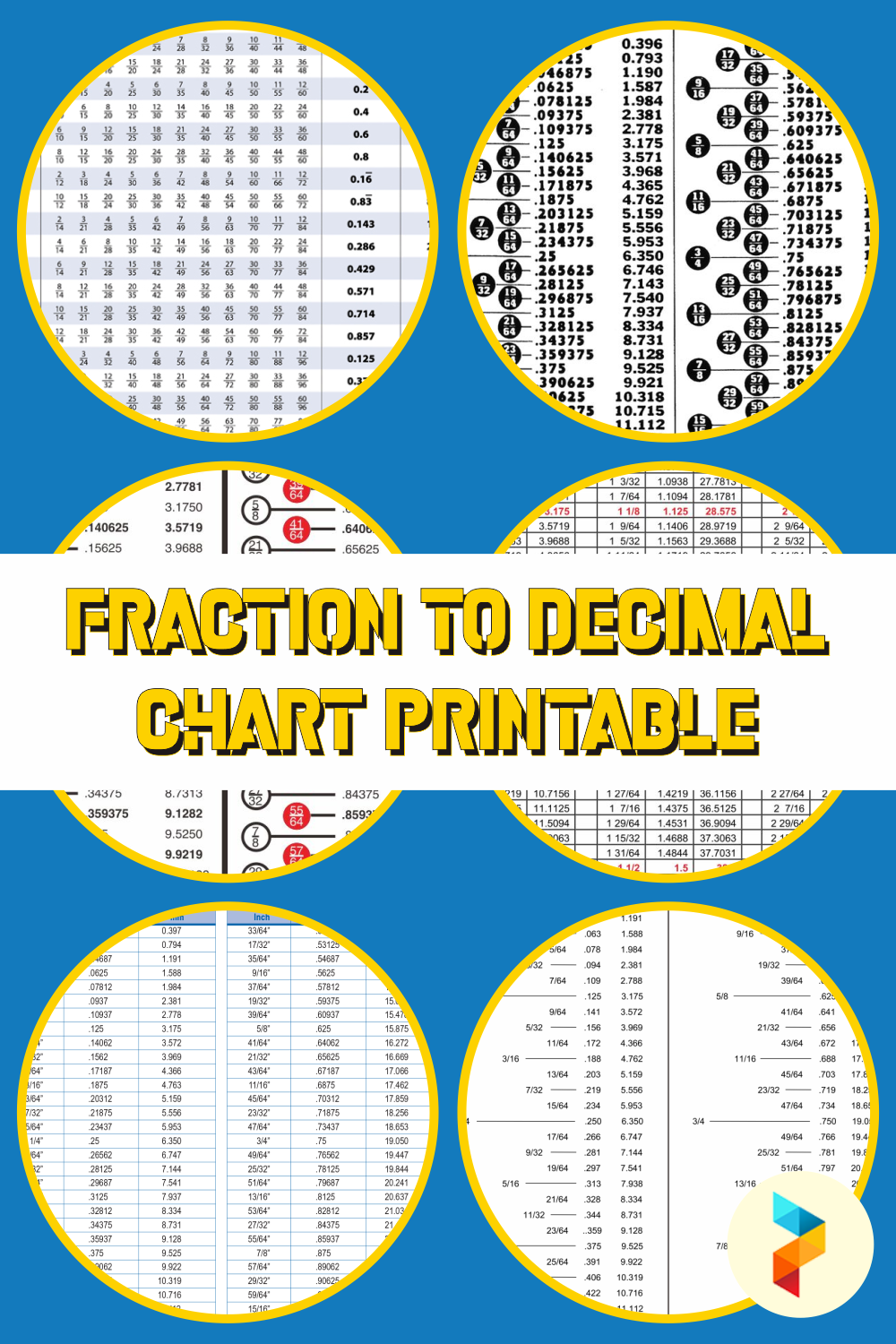### Table of Images 👆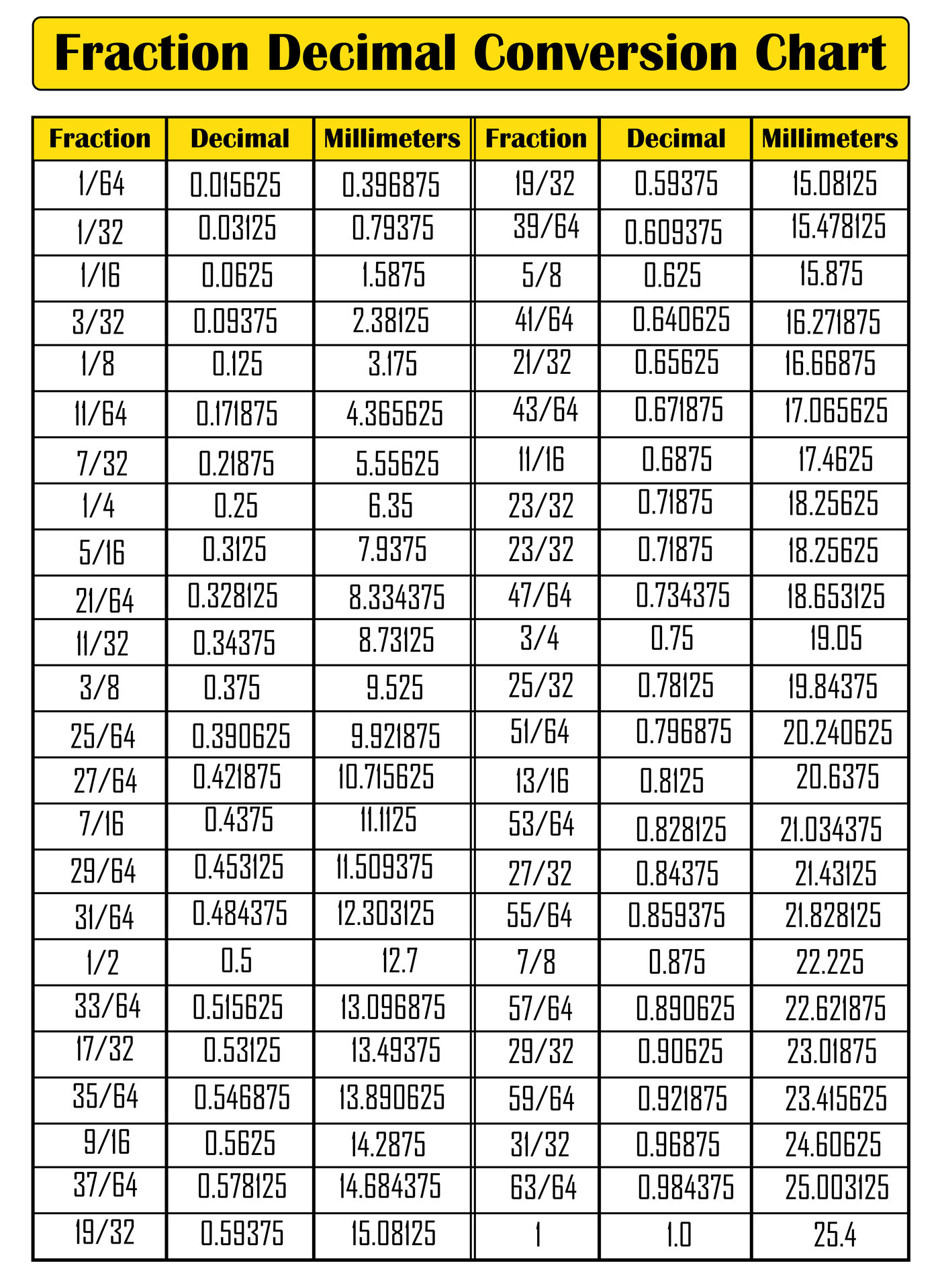## What is a Fraction?

In fact, the word fraction comes from the Latin word, fractus, which means "broken." So, fractions are part of a certain quantity. Fractions are used to write or read a quantity compared to the whole quantity. Therefore, a fraction is a number that represents an integer that has been divided into several parts.

If you study math, children will learn about these fractions. In mathematics, fractions are represented by a/b or read a per b. The number "a" is the quantifier and "b" is the denominator.

In general, fractions have 4 types. The four types of fractions are common fractions, decimal fractions, equivalent fractions, and mixed fractions.

• Common fractions: These are fractions that have two types, proper fractions, and improper fractions. A proper fraction is a fraction where the numerator is smaller than the denominator. Meanwhile, the improper fraction is the opposite of the true fraction.
• Decimal fractions: Decimal fractions have denominators that are multiples of 10 such as 10, 100, 100, and so on. It is written using a comma.
• Equivalent Fractions: These fractions have two or more other fractions with the same value ratio between the numerator and denominator.
• Mixed fractions: Mixed fractions can be obtained from improper fractions. This mixed fraction is a combination of integers and common fractions.

## Why Do We Need to Convert Fractions to Decimals?

To make it easier to calculate, fractions must be converted to decimals. Therefore, fractions and decimals are related to each other. A decimal number is the result of dividing the quantifier and denominator of the fraction.

However, the value of the quantifier must be less than the value of the denominator to get a decimal number. Apart from that, you can also change the denominator of these fractions to tens numbers so they can be easily converted to decimals.

## How to Convert Fractions to Decimals?

If you can teach how to convert fractions to decimals in a clear and fun way, children will easily understand the concept of fractions and decimals. Therefore, a step-by-step guide to converting fractions to decimals below can help you explain it to children.

• Understand the concept of converting fractions to decimals first and explain the concept to children. So, the conversion of a fraction to a decimal is done by dividing the quantifier by the denominator.

• Because you have to divide the quantifier and denominator, teach your children how to find the quantifier and denominator values of these fractions. In fractions (a/b), the quantifier is the number at the top (a) and the denominator is the number at the bottom (b).

• After that, start dividing the quantifier and denominator. This can be done using manual calculations or by using calculators. However, if the fractions are too difficult to calculate manually, you can teach children to use calculators. With those tools, children can still understand the concept of converting fractions to decimals but in an easier way. So, these calculators are only used to help children find the decimal value of fractions.

• Don't forget to teach them about commas in decimal numbers. So, once they have found the result, ask them to put a comma to get a decimal number.

• If you want to simplify decimal numbers, you can teach children to round off the last digit of the decimal number.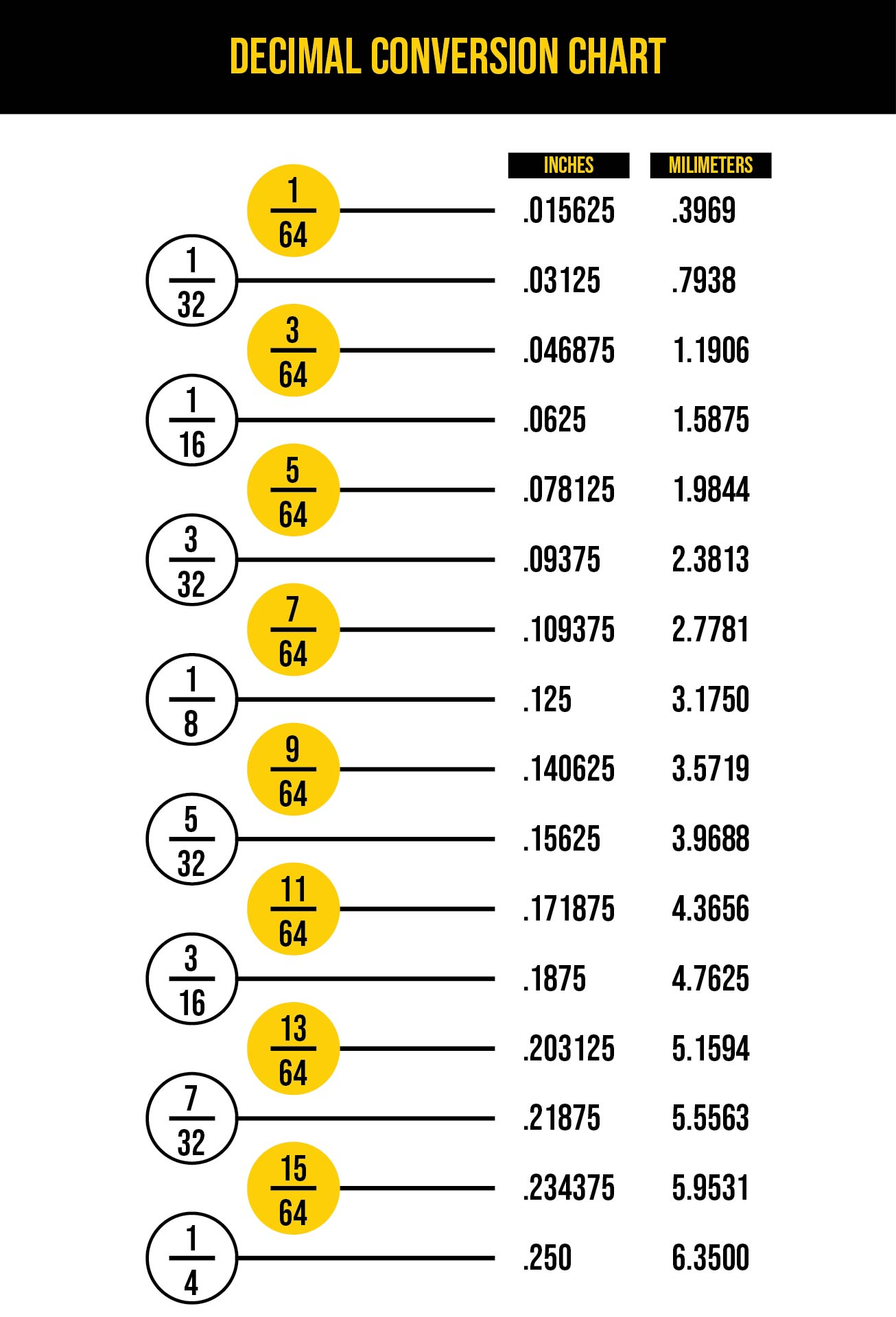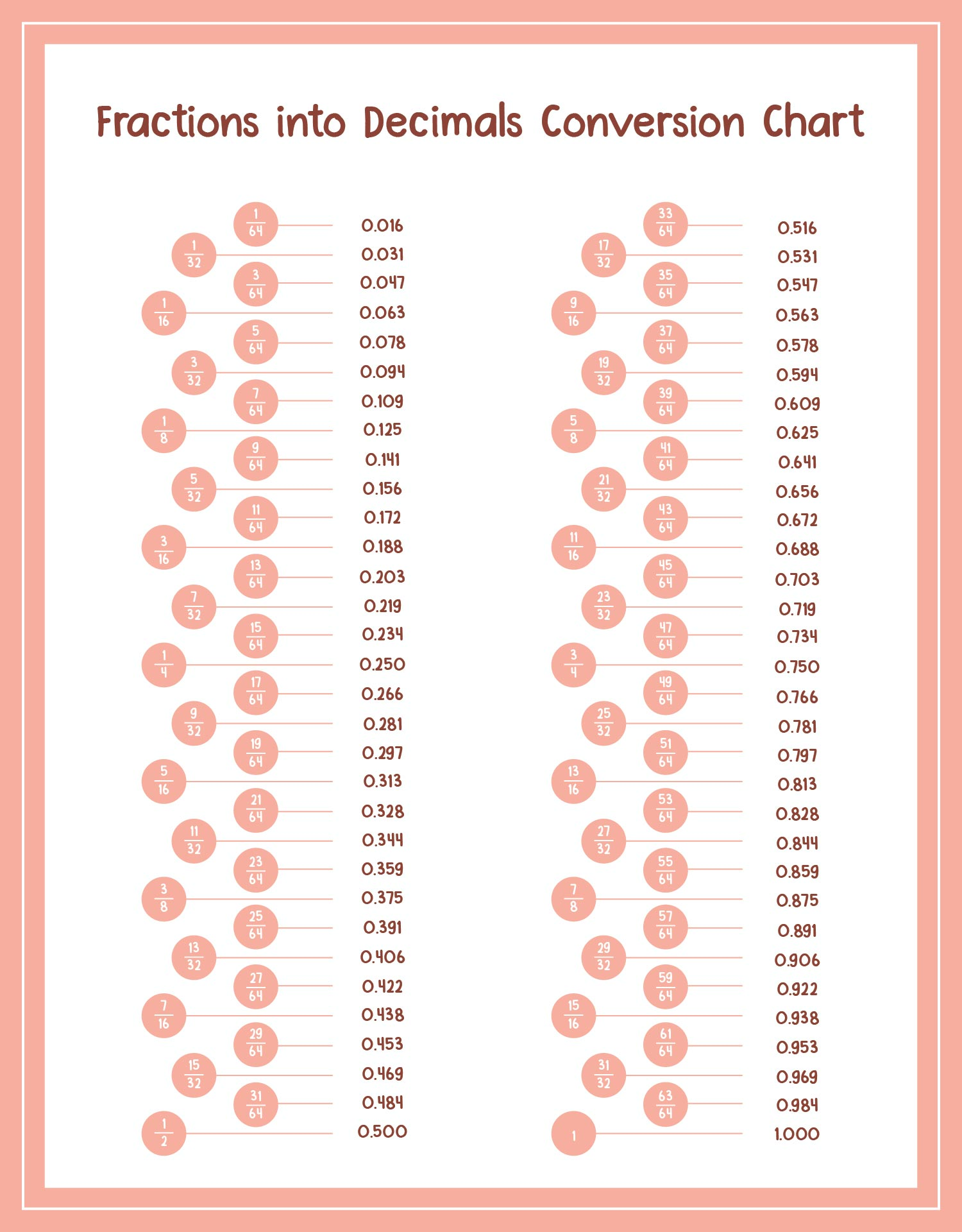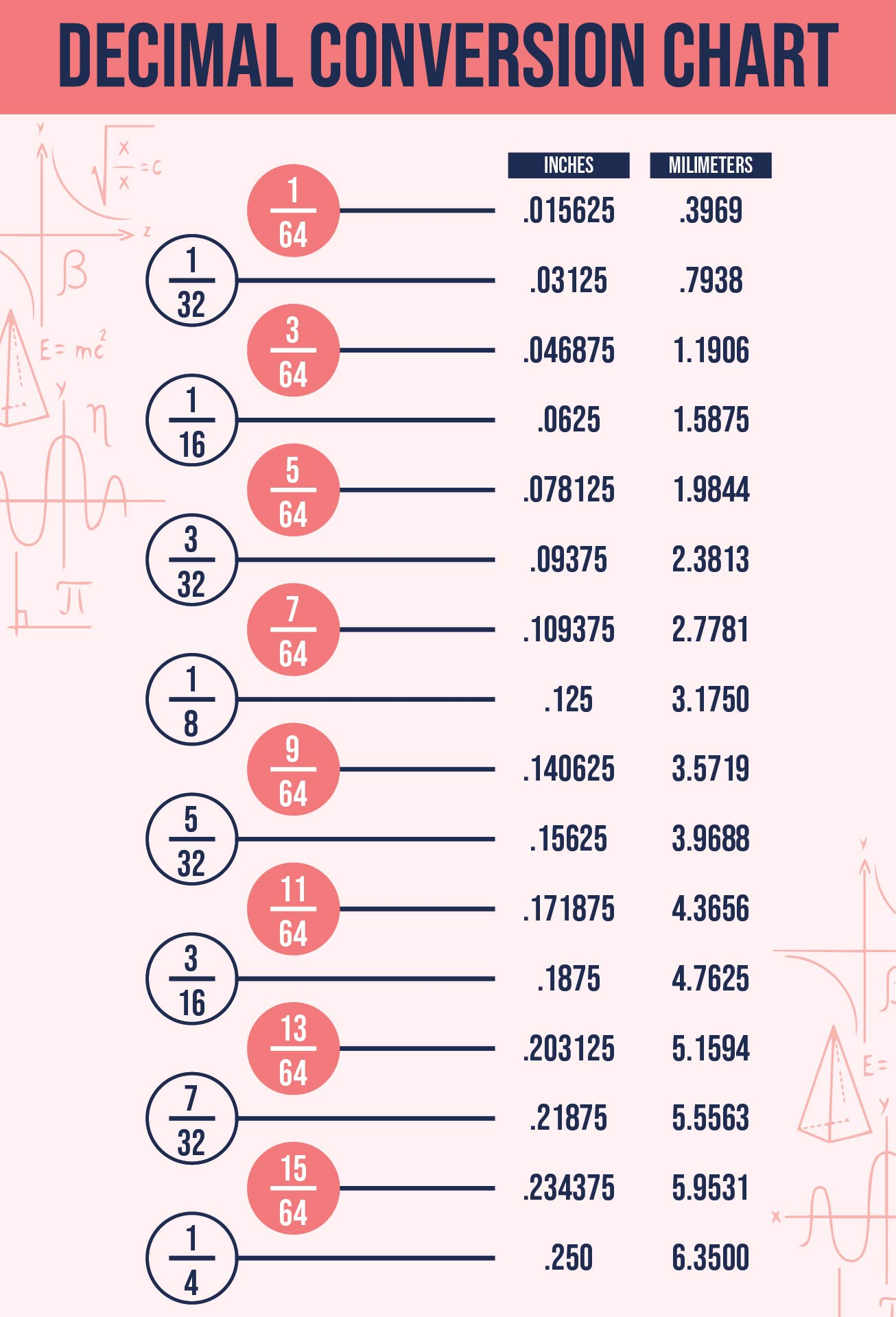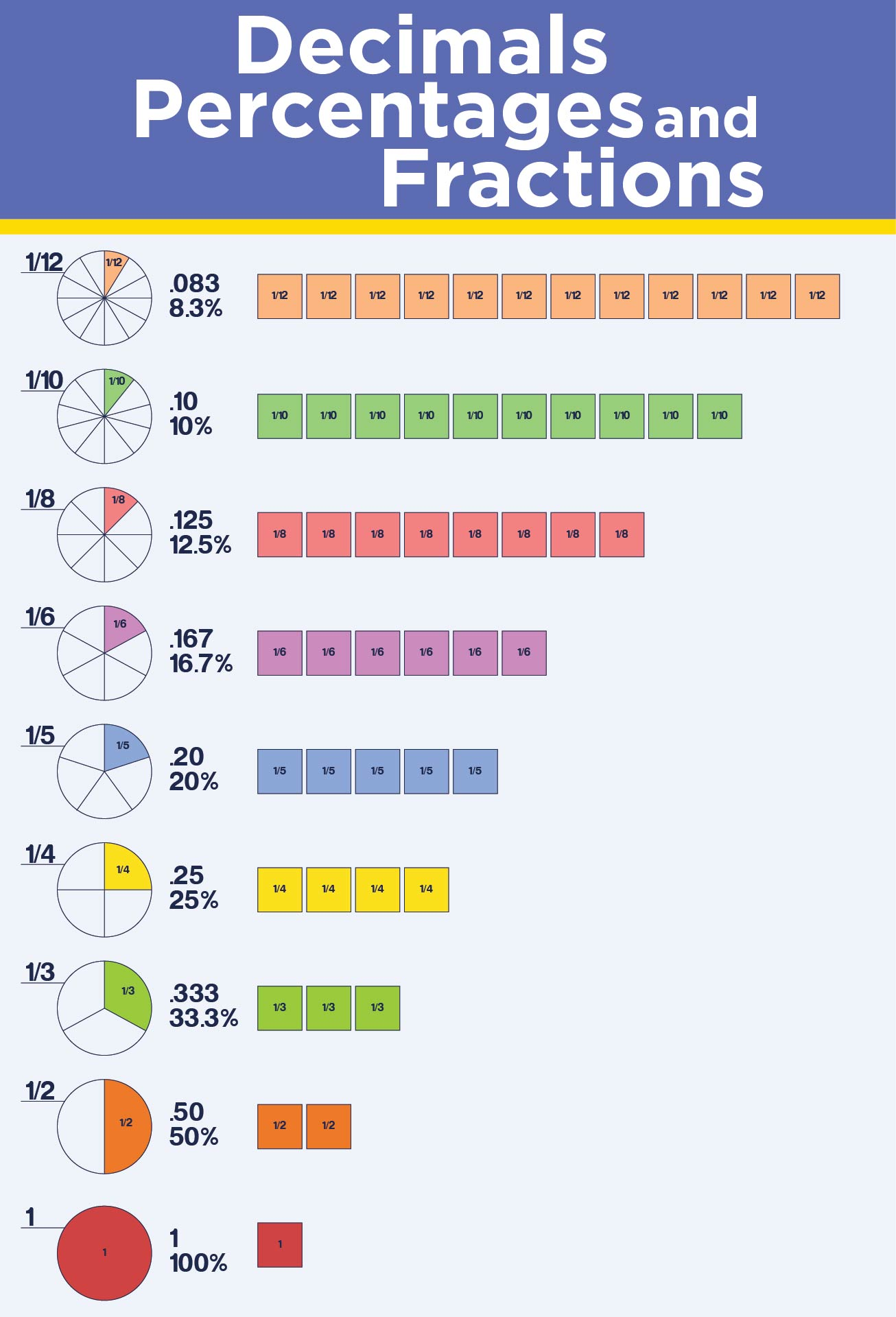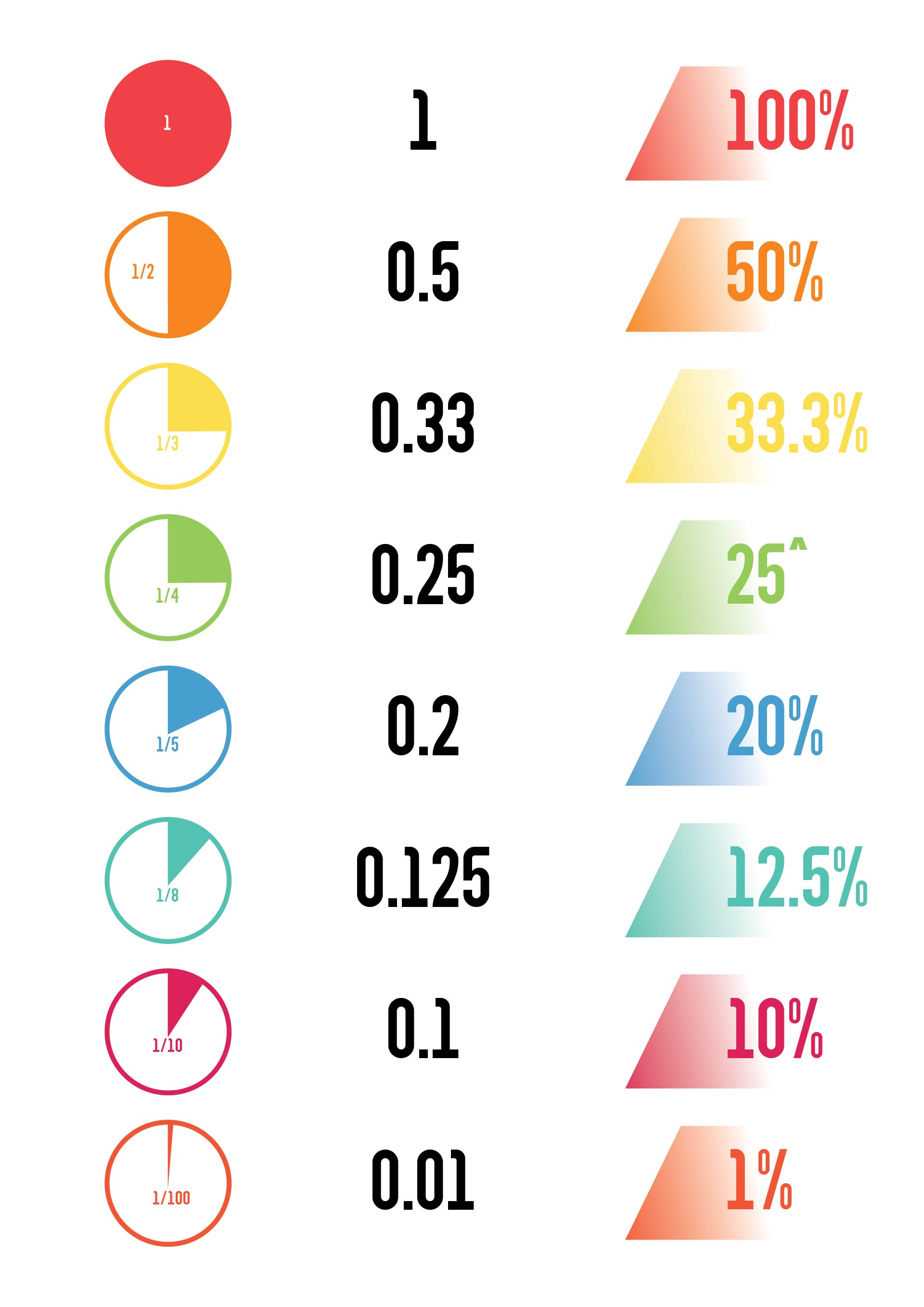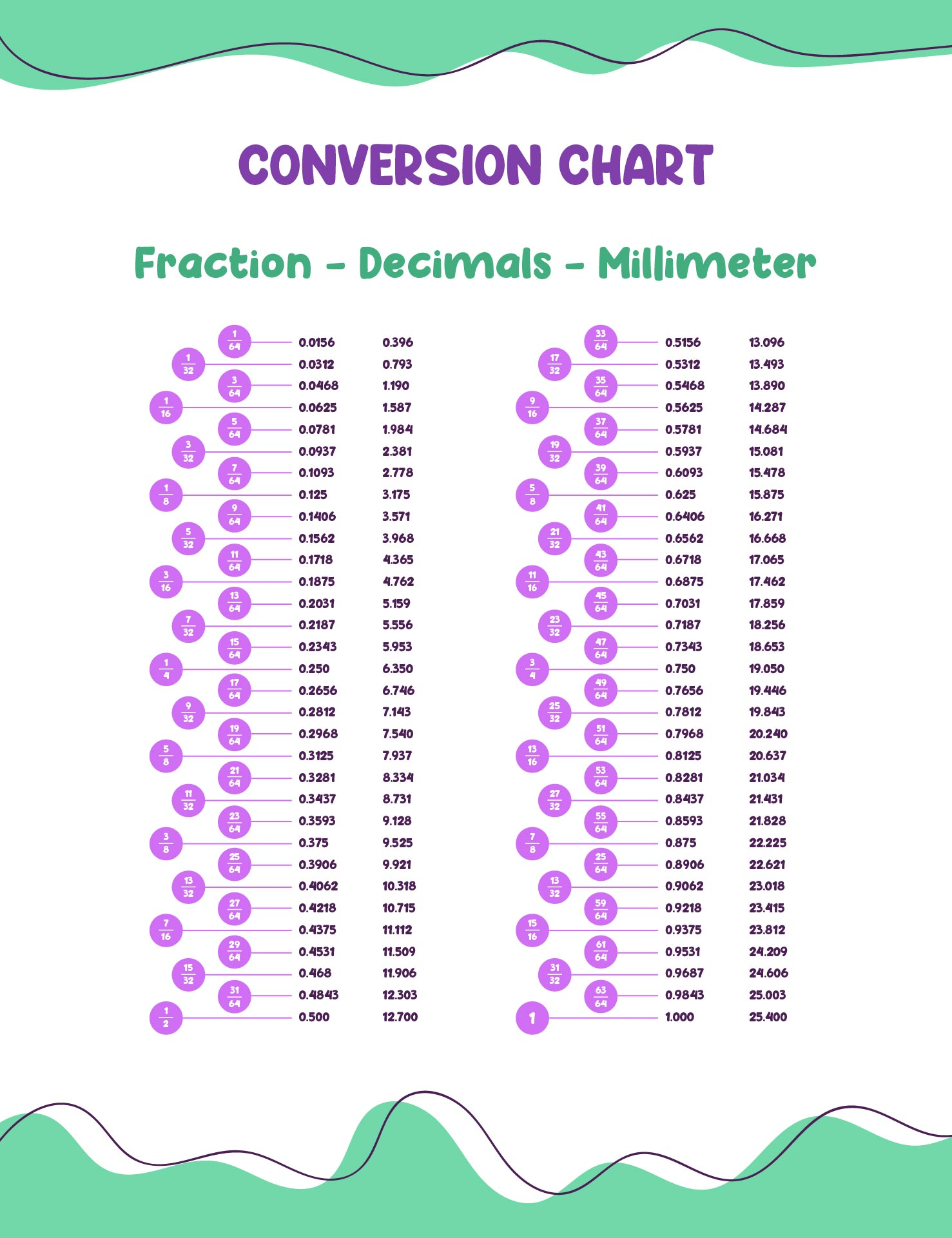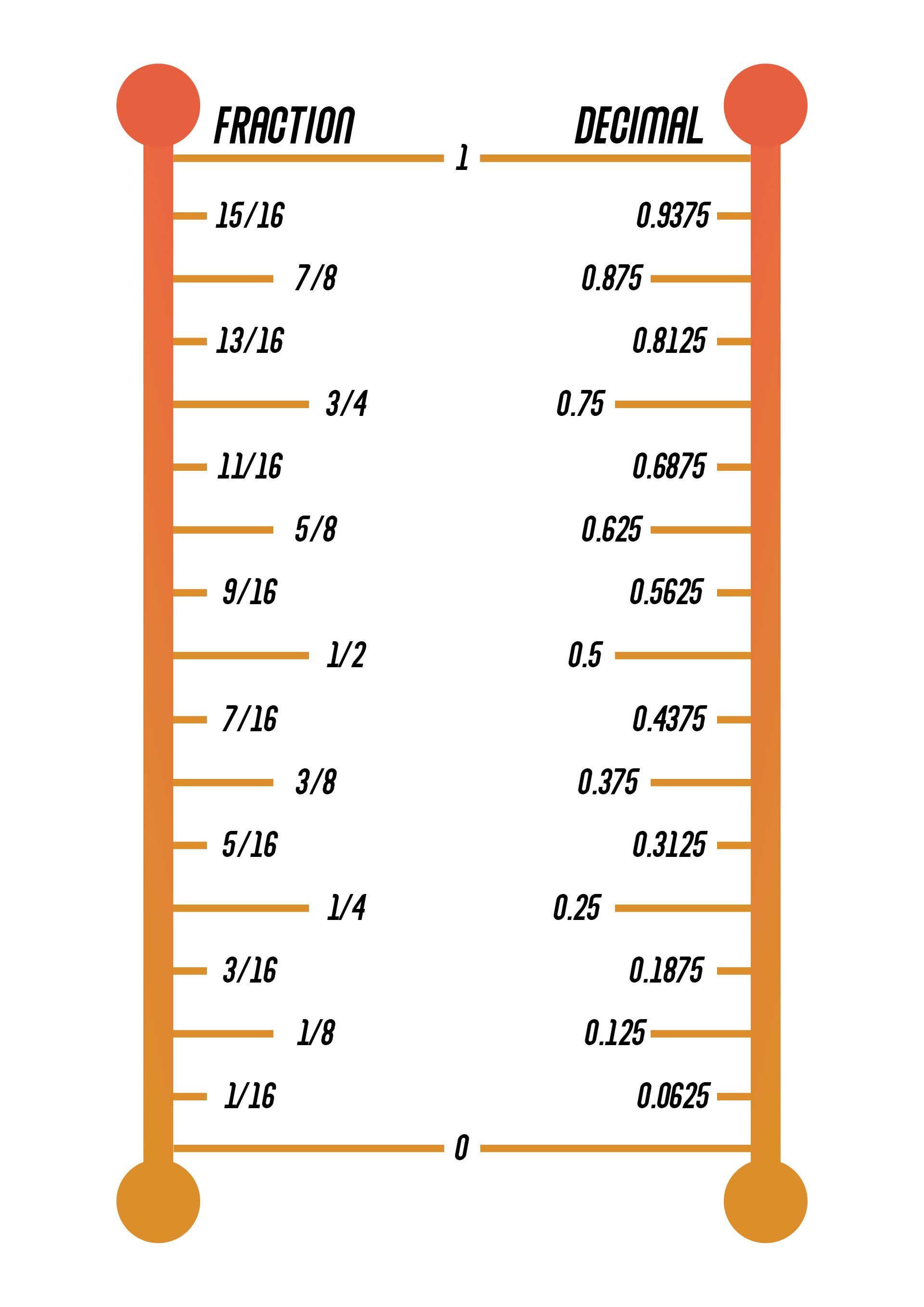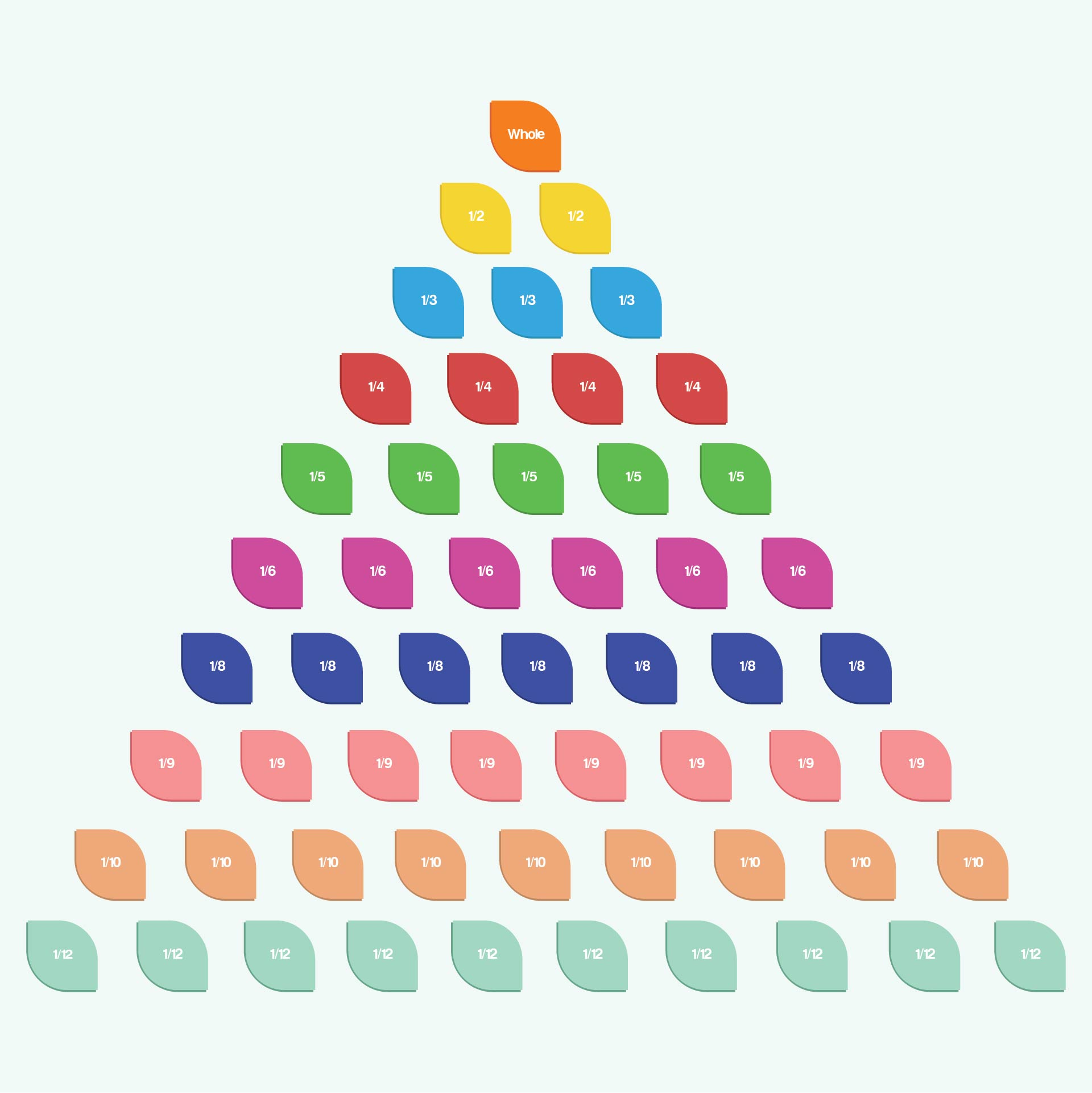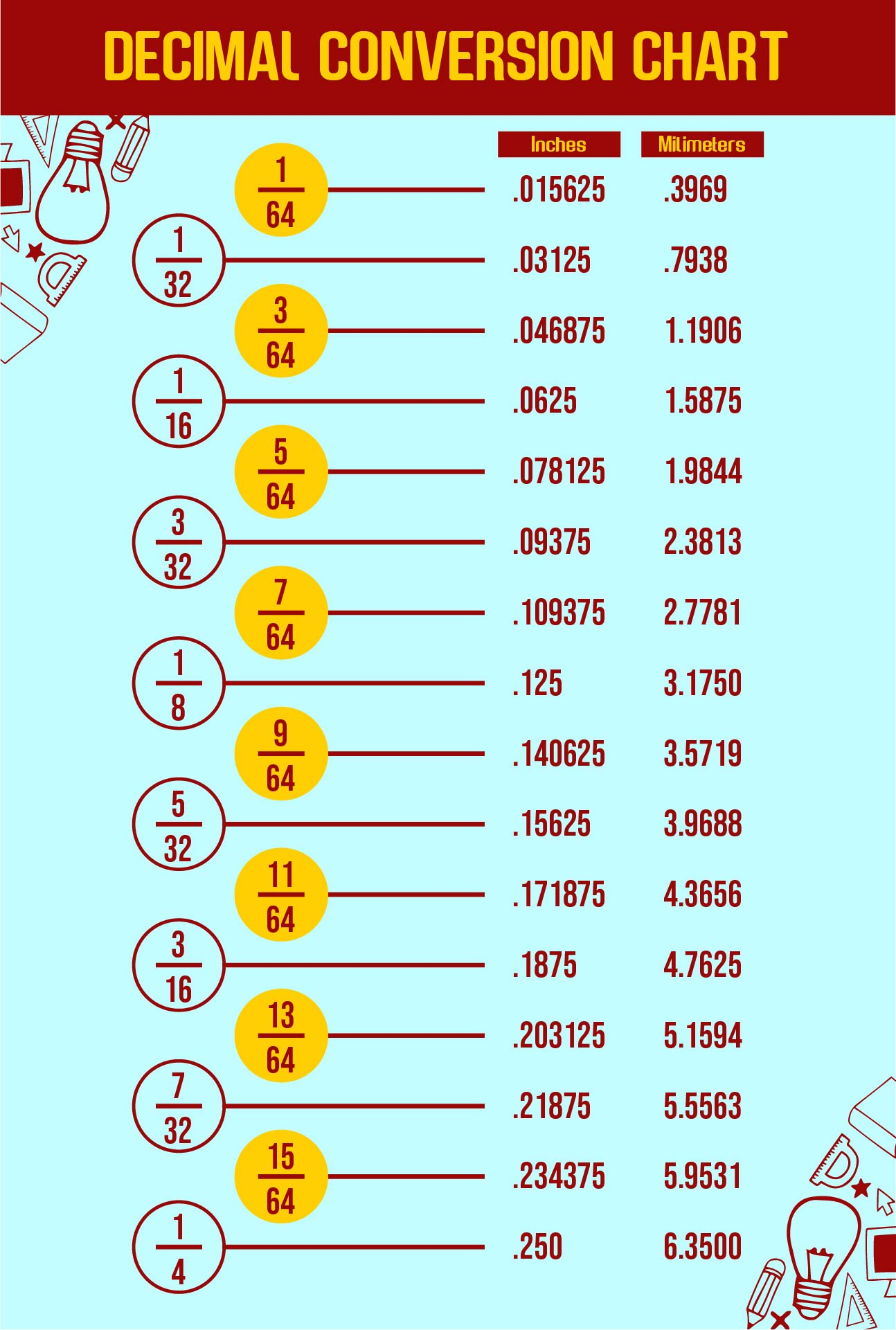## How to Explain the Concept of Decimals to Children?

As explained above, fractions must be converted to decimals to make the calculations easier. Well, teaching the concept of decimal numbers to children is a challenge. The reason is, the concept can be quite confusing for children. Therefore, apply these tips to explain the concept of decimals to children.

• First, explain the meaning of decimal numbers and their examples. Then, explain the position of the number in each of these decimal digits. It is better for you to write down the decimal number and explain the numbers in each position of the number. An example is 531. The 5 stands for hundreds, the 3 for tens, and the 1 for units.

• So, if the children already understand these basic concepts, you can explain that the decimal number is the number in the middle. Write a comma to the right of a number. After that, explain that the number written after the comma is the sign for the decimal number.

• Continue using an empty chart in the form of a box chart. On a 10-box chart, each square represents 0.1 or 1/10. Meanwhile, the 100 box represents 1/100 or 0.01 for each box. The function of the box diagram is to provide concrete proof to children that decimals are divisions or small parts of whole numbers. Don't forget to ask the children to color each of the boxes to show how much they add to their value.

• Still using the blank chart, you can also explain the relationship between decimal numbers and fractional numbers. Explain to them that decimal numbers are another way of representing fractions.

• Another way is to use a stick. So, you need to use a long stick and stick the number 1 on the right end of the stick. Meanwhile, the other end is numbered 0. Then, ask the children to write the number 0.5 in the middle of the stick. Next, ask the children to think about the decimal number in the interval.

Article written by Adelina M.K., last updated on Nov 10, 2022 and edited by Printablee Team.

#### More printable images tagged with:

Have something to tell us?

1.Valentina

A fraction to decimal chart printable is a useful tool that helps individuals easily convert fractions into decimals, making mathematical calculations more efficient and accurate.

2.Ainsley

The fraction to decimal chart printable is a useful tool for quickly and accurately converting fractions into decimals, making math calculations more efficient and accurate.

3.Adam

Great tool! This printable fraction to decimal chart is just what I needed to easily convert fractions to decimals. It's simple yet effective. Thanks!

4.Xavier

Great resource! This printable offers a quick and easy way to convert fractions to decimals. Very helpful for math practice!